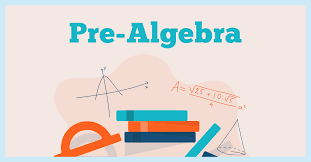Math 001

Pre Algebra

Prealgebra aims to improve essential math proficiency and problem solving skills to prepare students for Algebra and high school math curriculum. Topics include:

● Basic arithmetic operations and expressions

● Fractions, decimals, and percents

● Ratios and rates

● Exponents and square roots

● Simple number theory

● Variables and linear equations

● Negative numbers

● Basic geometry